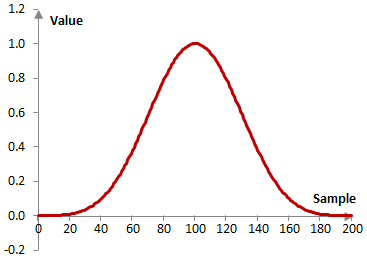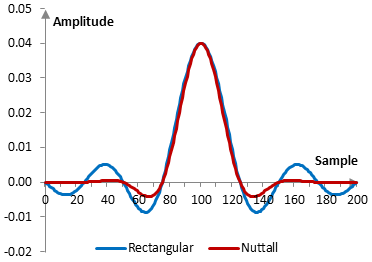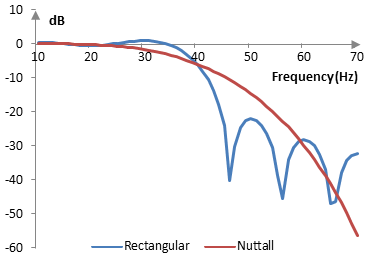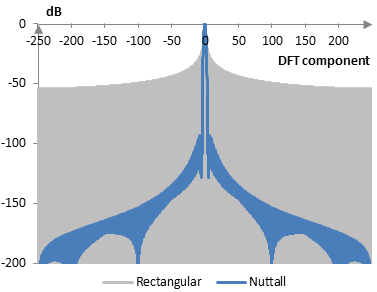# Nuttall window

The Nuttall window coefficients are given by the following formula

$$a(k)= 0.355768 - 0.487396 \, \cos(\frac{2 \pi k}{N-1}) + 0.144232 \, \cos(\frac{4 \pi k}{N-1}) - 0.012604 \, \cos(\frac{6 \pi k}{N-1})$$

where N is the length of the filter and k = 0, 1, …, N – 1.

Consider a finite impulse response (FIR) low pass filter of length N = 201. The following is the Nuttall window.Given a sampling frequency of 2000 Hz and a filter cutoff frequency of 40 Hz, the impulse response of the filter with a rectangular window (with no window) and with the Nuttall window is as follows.The magnitude response of the same filter is shown on the graph below.## Measures for the Nuttall window

The following is a comparison of the discrete Fourier transform of the Nuttall window and the rectangular window.The Nuttall window measures are as follows.

 Coherent gain 0.36 Equivalent noise bandwidth 2.03 Processing gain -3.06 dB Scalloping loss -0.81 dB Worst case processing loss -3.87 dB Highest sidelobe level -93.3 dB Sidelobe falloff -23.4 dB / octave, -77.6 dB / decade Main lobe is -3 dB 1.92 bins Main lobe is -6 dB 2.68 bins Overlap correlation at 50% overlap 0.035 Amplitude flatness at 50% overlap 0.423 Overlap correlation at 75% overlap 0.452 Amplitude flatness at 75% overlap 1.000

Window

### Filtered HTML

• Freelinking helps you easily create HTML links. Links take the form of [[indicator:target|Title]]. By default (no indicator): Click to view a local node.